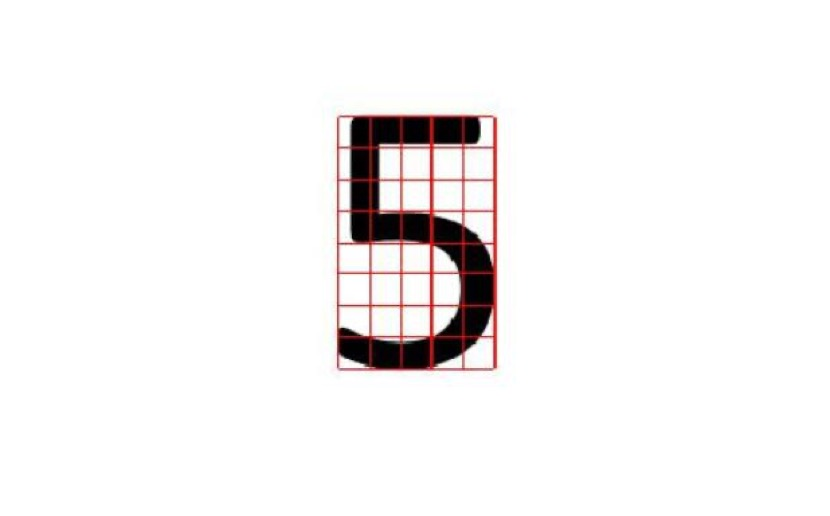# 仿真系统搭建

### 1 系统框图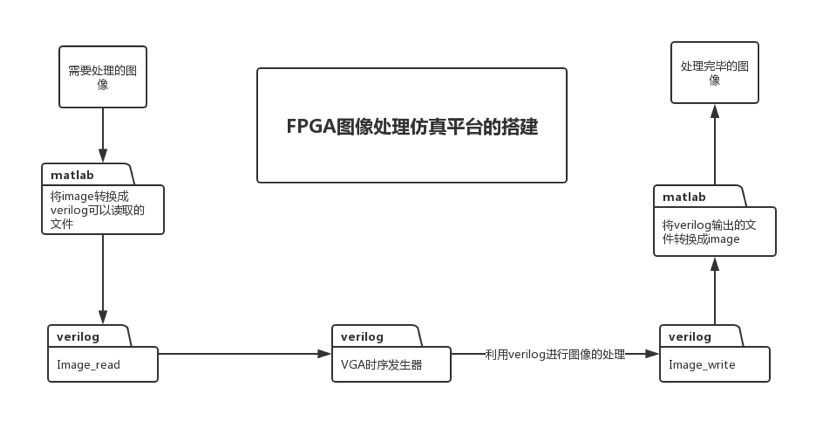### 2 VGA 时序发生器

`define pix_800_600

module vga_ctl

(

input pix_clk,

input reset_n,

input [23:0] VGA_RGB,

output VGA_CLK,

output [11:0] hcount,

output [11:0] vcount,

output [7:0] VGA_R,

output [7:0] VGA_G,

output [7:0] VGA_B,

output VGA_HS,

output VGA_VS,

output VGA_DE,

output BLK

);

`include “vga_parameter.vh”

`ifdef pix_800_600

​ //———————————//

​ // 800*600 60HZ pixel clock 40.00MHZ

​ //——————————–//

​ parameter H_Total = 1056;

​ parameter H_Sync = 128;

​ parameter H_Back = 88;

​ parameter H_Active = 800;

​ parameter H_Front = 40;

​ parameter H_Start = 216;//H_Sync+H_Back

​ parameter H_End = 1016;//H_Sync+H_Back+H_Active

​ //——————————-//

​ // 800*600 60HZ

​ //——————————-//

​ parameter V_Total = 628;

​ parameter V_Sync = 4;

​ parameter V_Back = 23;

​ parameter V_Active = 600;

​ parameter V_Front = 1;

​ parameter V_Start = 27;//V_Sync+V_Back

​ parameter V_End = 627;//V_Sync+V_Back+V_Active

`endif

## 3图像数据转换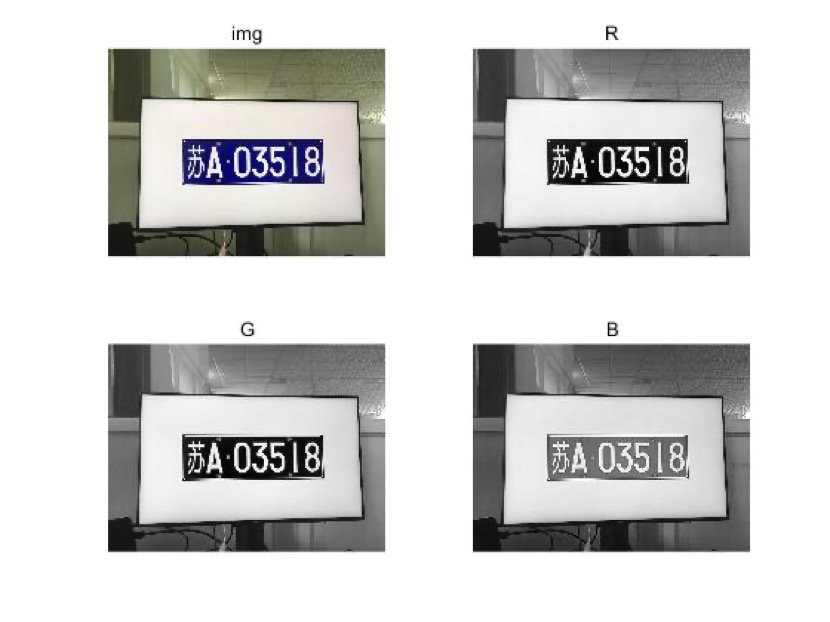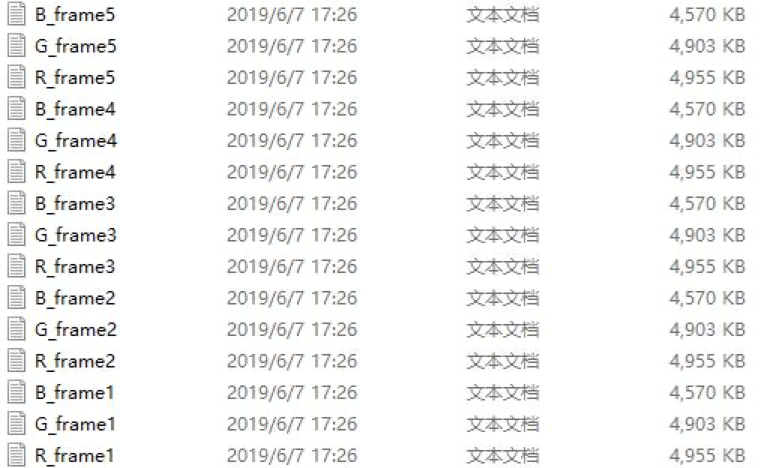`timescale 1ns/1ps

`define NULL 0

input pixel_clk,

input reset_n,

input de,

input [11:0] frame_cnt,

output [23:0] rgb

);

`timescale 1ns/1ps

`define NULL 0

module imwrite_frame4(

input pixel_clk,

input reset_n,

input de,

input [11:0] frame_cnt,

input [23:0] rgb

);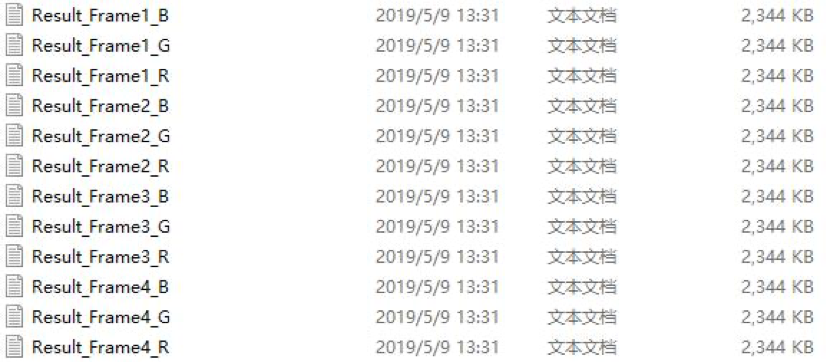)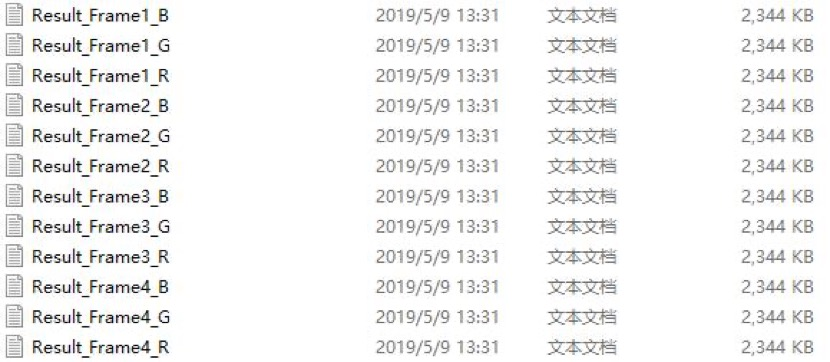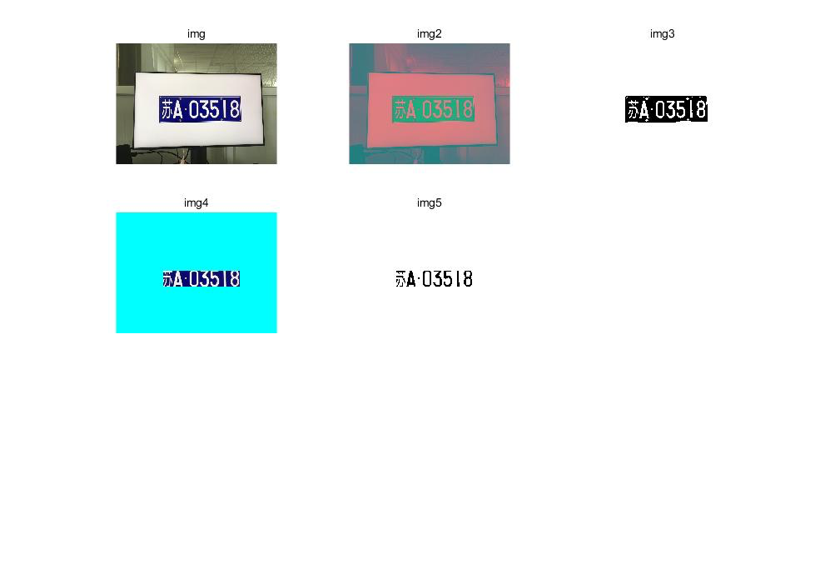# 图像处理仿真

## 1 RGB 转 YCbCr

​ 为了方便进行图像阈值的提取，利用 verilog 实现了 RGB 到 YCbCr 的色域转换，计算式可以百度: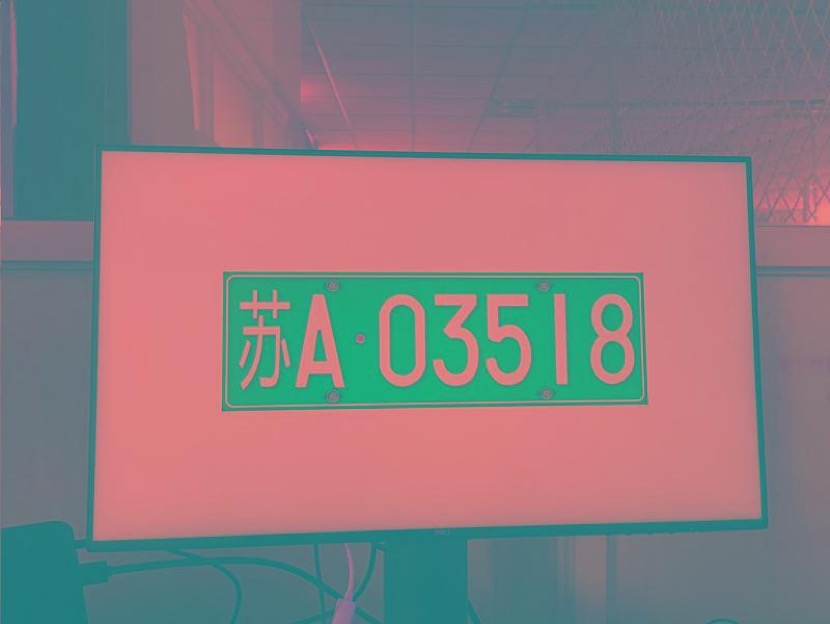rgb2ycbcr U_rgb2ycbcr(

.pixelclk(pixel_clk),

.i_rgb(VGA_RGB),

.i_hsync(VGA_HS),

.i_vsync(VGA_VS),

.i_de(VGA_DE),

.o_rgb(y_rgb),

.o_ycbcr(y_ycbcr),

.o_gray(y_gray),

.o_hsync(y_o_hsync),

.o_vsync(y_o_vsync),

.o_de(y_o_de));

### 2 阈值确定与二值化

​ 首先将车牌从复杂的环境中提取出来。确定车牌的阈值，二值化。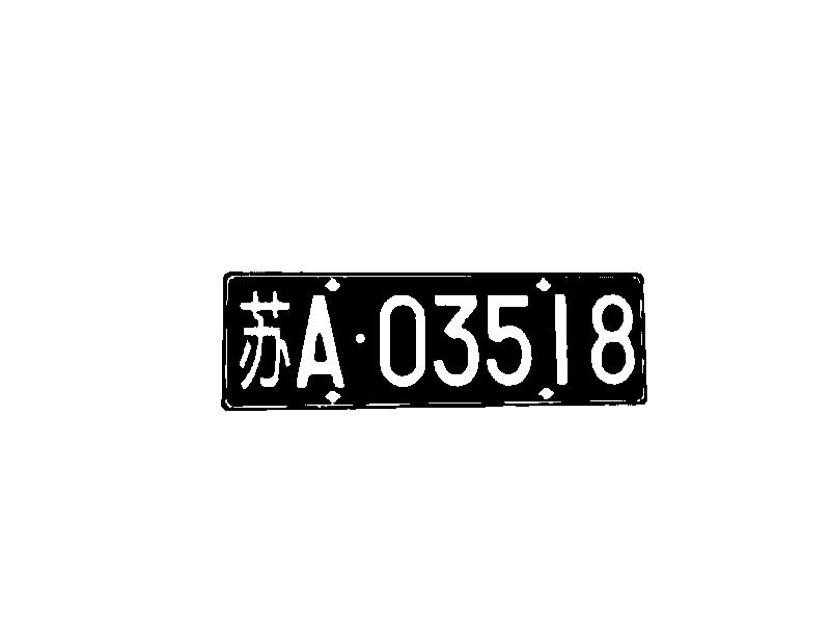ycbcr_location#( //Paramter Define such as threshold

​ )U_ycbcr_location(

​ .pixelclk(pixel_clk),

​ .reset_n(reset_n),

​ .i_rgb(y_rgb),

​ .i_ycbcr(y_ycbcr),

​ .i_gray(y_gray),

​ .i_hsync(y_o_hsync),

​ .i_vsync(y_o_vsync),

​ .i_de(y_o_de),

​ .binary_image(yl_binary),

​ .rgb_image(yl_rgb),

​ .gray_image(yl_gray),

​ .o_hsync(yl_o_hsync),

​ .o_vsync(yl_o_vsync),

​ .o_de(yl_o_de)

​ );

## 3 锁定车牌位置

​ 通过水平垂直投影，可以将车牌框选出来，并且为了便于识别数字，我们将车牌以外的颜色转换成近似车牌的底色，便于进行第二次的垂直投影与阈值提取。此外，框选车牌的同时，削掉了一部分上下边界，去除了定孔。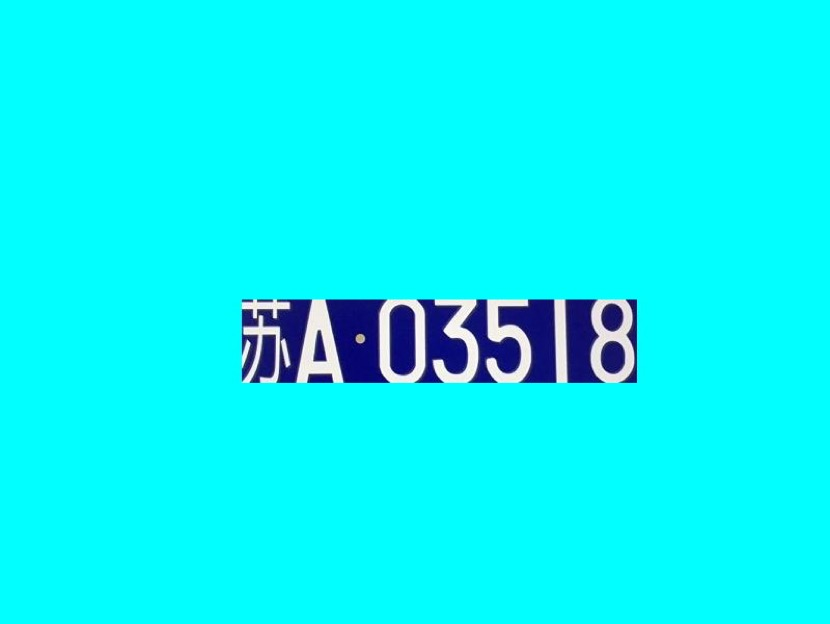Vertical_Projection#( //Paramter Define such as threshold

)U_Vertical_Projection(

.pixelclk(pixel_clk),

.reset_n(reset_n),

.i_binary(HV_dout),

.i_hs(HV_o_hsync),

.i_vs(HV_o_vsync),

.i_de(HV_o_de),

.i_hcount(hcount),

.i_vcount(vcount),

.hcount_l(hcount_l),

.hcount_r(hcount_r),

.vcount_l(vcount_l),

.vcount_r(vcount_r));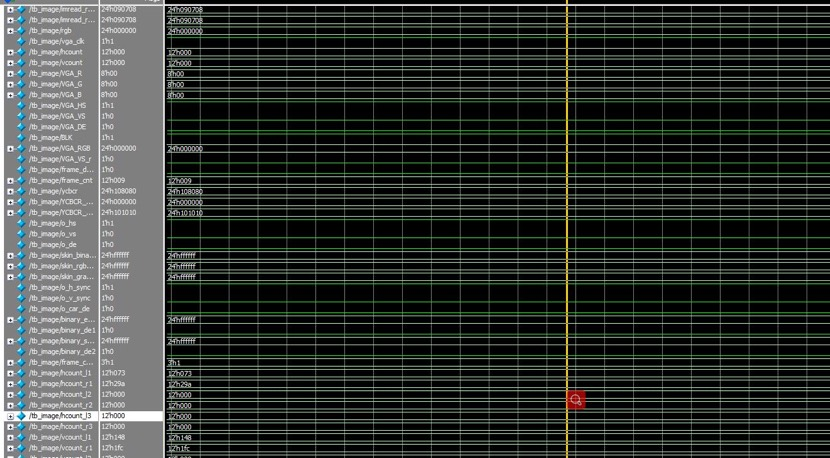## 4 提取车牌数字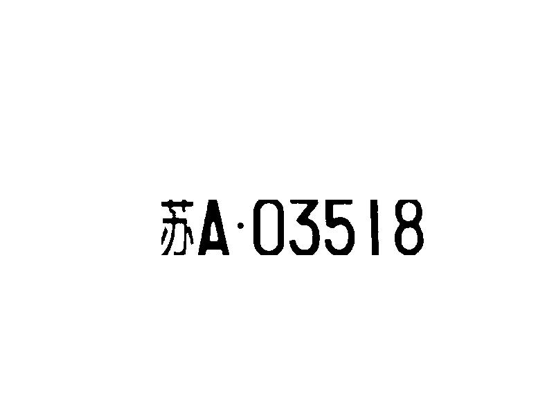## 5 水平垂直投影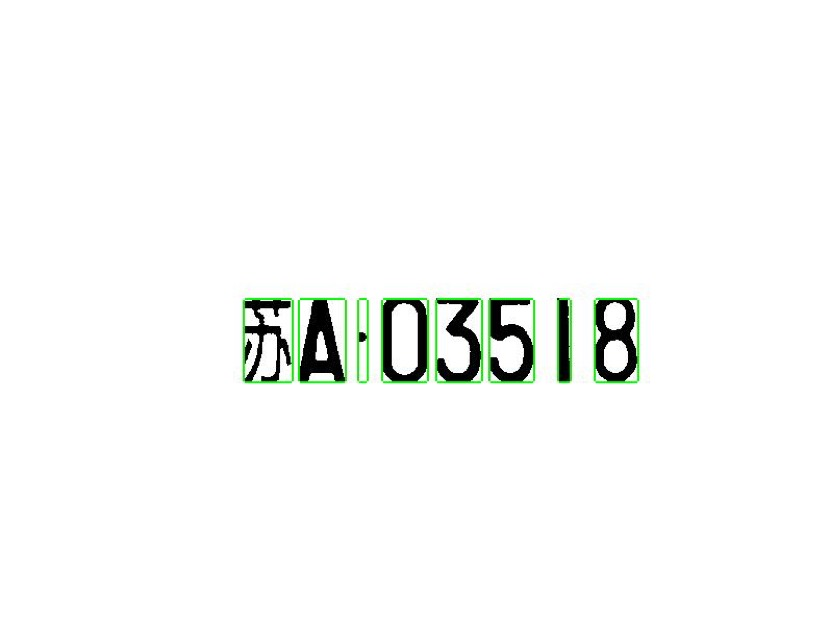## 7 方案二:5X8 维矩阵检测